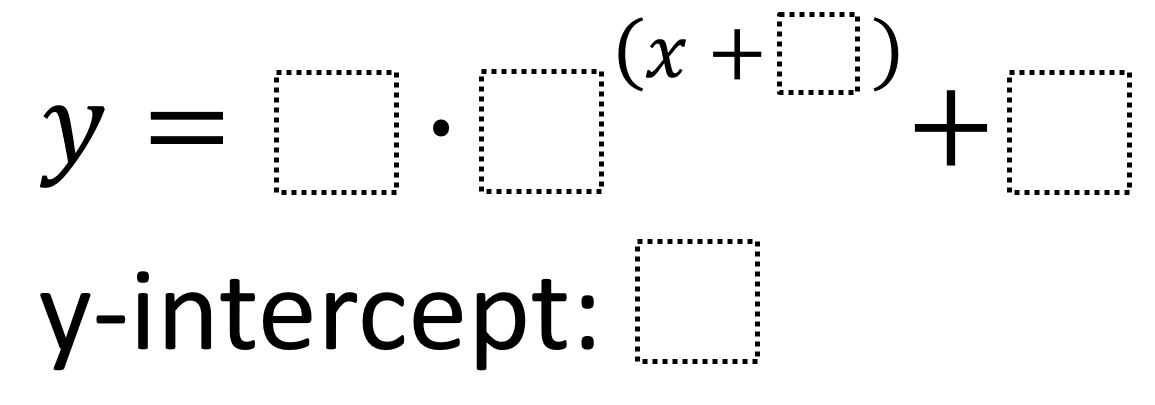# Exponential Function Features 2

Directions: Use the integers -9 to 9, at most two times each, fill in the boxes to create an exponential growth function with the greatest possible y-intercept.### Hint

How do each of the values you can choose affect the values of the y-intercept?

The greatest possible y-intercept is 9 and can be gotten through an equation like:

y = 4 * 2^(x+0) + 5
y-intercept: 9

Source: Robert Kaplinsky

## Asymptotes of Rational Functions

Directions: Using the digits 1 to 9 at most one time each, place a digit …

1.•